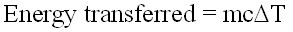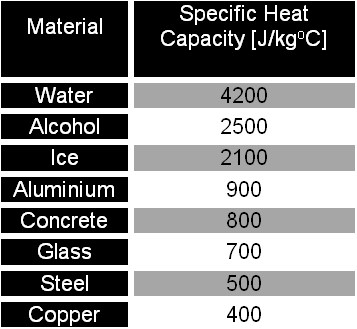# Heat Capacity and Nature Of Substance# Heat Capacity

Matter becomes hotter when heat is transferred to them. This energy is absorbed by the molecules which result in the molecules gaining kinetic energy, hence moving faster. The faster moving molecules cause the temperature of the system to rise. These kinetic energies of individual molecules, together with the potential energies that come from the intermolecular forces, are collectively called the internal energy of the system.

When a system is heated up, its molecules gained energy. If the mass of the system is large, then the heat supplied will be distributed to more particles, which result in less energy changes per particle. This means that the temperature of the system will not rise much because the temperature rise is related to the energy supplied to the system.

This relationship between the energy supplied, mass and the temperature change can be expressed in the formula:whereis the energy supplied,is the mass of system,is the temperature change andis the specific heat capacity of the material. The SI unit of specific heat capacity is J/kg. The specific heat of some common materials are show in the table below.

Materials Specific heat capacity / J kg-1K-1
Pure water 4200
Copper 390
Aluminium 910
Stainless steel 510

We shall discuss the physical meaning of thermal capacity.

Consider the situation when we have a 100 g of copper and a 1 kg copper block. Itis common sense that the 1 kg will need to absorb more heat in order to increase its temperature by the same amount compared to the 100 g copper block. We say that the 1 kg copper block has a larger thermal capacity than the 100 g block, even though they are made from the same material. If we have 2 blocks of copper of same mass, we can see that they will absorb the same amount of heat to rise the same temperature. Thus, two objects made of same material and having same mass will have the same thermal capacity. If we are to standardize this mass to 1 kg, then we can say that the two objects have the same specific thermal capacity, or specific heat capacity.Consider another situation. We have two blocks, one made of 1 kg of copper and the other made of 100 g of aluminium. The copper block needs to absorb more heat than the 100 g aluminium block in order to rise the same temperature. We can say that the copper block have a higher thermal capacity than the aluminium block. However, we can see that this is not a fair comparison as the copper has a larger mass. If we standardize the alunimium to 1 kg, we can see that it now requires more heat than the 1 kg copper. Thus, the aluminium has a higher specific heat capacity than copper.

Specific Heat Capacity

_____________________________________________________________________________________

## What is Specific Heat Capacity?

Specific Heat Capacity is the amount of energy (in Joules) needed to increase the temperature of 1 kg of a substance by 1 degree Celsius. The higher the value the more energy that needs to put in. Using this information, it is possible to calculate how much energy needs to be out into a substance to increase it temperature by a specific amount.

The equation for this is: energy transferred = mass x specific heat capacity x temperature change

This question will most likely to appear in the exam, so make sure you learn this equation!

## The symbols for the above equation are as follows:## Specific Heat Capacity ChartApplications

Because water has a high specific heat capacity, it is a useful substance for storage and carrying thermal energy, such as in central heating systems. It is also used in cooling systems in cars; the water removes unwanted thermal energy to the radiator from the engine.

Latent Heat

Latent heat is the amount of energy that has to be transferred to 1 kg of a substance to change its state. Latent Heat is the amount of energy that is realised or absorbed by a substance in the form of heat when it is changing state. The two main types of latent heat are latent heat of fusion (melting substance) and latent heat of vaporisation (boiling substance). An example of latent heat fusion is an ice cube at room temperature. When the ice cube is melting, it is absorbing energy in the form of heat but its temperature does not change, the energy absorbed is called the latent heat of fusion.

The equation for working out the amount of energy released from a substance with a known mass goes as follows:

Energy transferred = mass x specific latent heat

Energy transferred = mL

Latent Heat of Fusion of Ice: 330,000J/Kg

Latent Heat of Vaporisation of Water: 2,300,000J/Kg